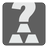How Much Gold
Simple
English FR RU

Our Favorite Trio has found a mysterious metal bar. This bar appears to be made of various metal including gold, iron, copper and tin. The bar does not contain any other metal except these. We do not know the quantity of each metal in the bar, but we do know the proportions of the various alloys. For example: the gold and tin proportion is 1/2, gold and iron -- 1/3, gold and copper -- 1/4. "the gold and tin proportion is 1/2" means that gold and tin together (their sum, not their ratio!) are the 1/2 of the whole bar (the sum of them all). You should calculate the proportion of gold in the entire bar.

The proportions are given as a dictionary where keys are alloy names and values are proportions. The alloy names are presented as strings, which are composed of two metal names separated by a dash (Ex: "gold-tin", "iron-copper", "iron-gold"). The proportions are presented as fractions (fractions.Fraction date type. Read about this module).You should return the proportion of gold in the bar as a fraction (fractions.Fraction).

Input: Alloys names and proportions as a dictionary.

Output: Proportion of gold as a fractions.Fraction.

Example:

```checkio({
'gold-tin': Fraction(1, 2),
'gold-iron': Fraction(1, 3),
'gold-copper': Fraction(1, 4),
}) == Fraction(1, 24)
checkio({
'tin-iron': Fraction(1, 2),
'iron-copper': Fraction(1, 2),
'copper-tin': Fraction(1, 2),
}) == Fraction(1, 4)```

How it is used: This task teaches you how to work with the Fraction data type. Fraction is very useful data type when you work with fractional numbers and want to avoid the float-rounding problem. You can learn how to use equation sets and pick out information from the combined data.

Precondition:
All tests are solvable.
The bar contains all four metals.